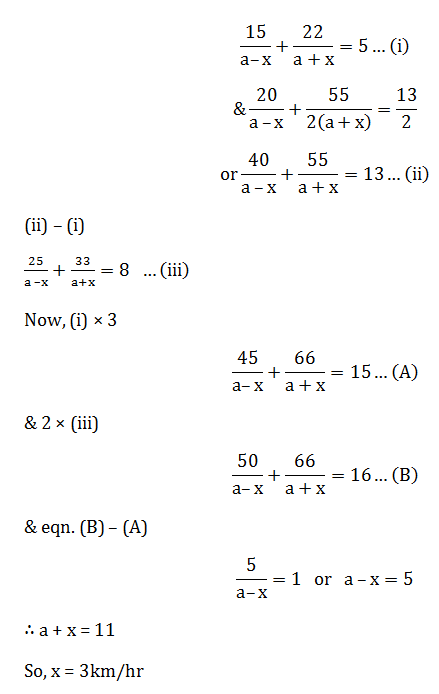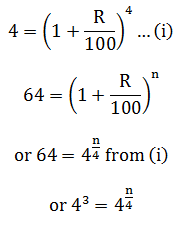# SSC Quantitative Aptitude Questions (Day-55)

Dear Aspirants, you can find the Quantitative Aptitude questions with detailed explanations for the SSC exams. Nowadays the competitive level of the exam has been increasing consistently. Due to the great demand for the government job, the level of the toughness reached greater. Candidates have to enhance the preparation process in order to drive in the right path. It doesn’t need to clear the prescribed cutoff. You must have to score good marks more than the cut off marks to get into the final provisional list. Here we have updating the Quantitative Aptitude questions with detailed explanations on a daily basis. You can practice with us and measure your level of preparation. According to that you can sculpt yourself in a proper way. SSC aspirants kindly make use of it and grab your success in your career.

Start Quiz

1) Three bottles of equal capacity are containing a mixture of milk and water in ratio 2 : 1, 3 : 7 and 4 : 11 respectively. These three bottles are emptiedinto a large bottle. What is the ratio of milk and water respectively in this large bottle?

(a) 37 : 53

(b) 37 : 90

(c) 37 : 30

(d) 7 : 30

2) The average of 11 results is 182. If the average of first 6 results is 199 and that of the last 6 results is 161, then what will be the 6th result?

(a) 79

(b) 118.5

(c) 158

(d) 237

3) The average of 45 results was calculated as 27 but later it was found that while calculating 39 was taken as 93 by mistake, then what will be the correctaverage?

(a) 25.8

(b) 26.8

(c) 27.2

(d) 28.2

4)  A shopkeeper professes to sell his goods at cost price but uses a 960 gm weight instead of 1 kilogram weight. What is the profit percentage of theshopkeeper?

(a) 4 1/6

(b) 6 1/4

(c) 5 1/7

(d) 5 1/6

5) A person sold a book for Rs 21 and got a loss percentage which was numerically equal to the cost price. What is the cost price (in Rs) of the book?

(a) 30

(b) 70

(c) Both 30 and 70

(d) Cannot be determined

6) If length of a rectangle is increased by 10% and breadth is increased by 15%, then what will be the percentage increase in the area of rectangle?

(a) 25.5

(b) 25

(c) 28.4

(d) 26.5

7) If the base of triangle is increased by 10% and height is decreased by 20%, then what will be the percentage change in the area of a triangle?

(a) 30

(b) 20

(c) 22

(d) 12

8) A bus starts running with some initial speed and its speed increases every hour by 9 km/hr. If it takes 11 hours to cover a distance of 572 km, thenwhat was the initial speed (in km/hr) of the bus?

(a) 3.5

(b) 7

(c) 10.5

(d) 14

9) A boat goes 15 km upstream and 22 km downstream in 5 hours. It goes 20 km upstream and
55/2 kmdownstream in 13/2 hours. What is the speed (in km/hr) of stream?

(a) 3

(b) 5

(c) 8

(d) 11

10) If a certain sum becomes 4 times in 4 years at compound interest, then in how many years, it will become 64 times?

(a) 5

(b) 12

(c) 16

(d) 24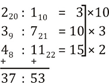∑11 = 11 × 182

∑6₁ = 199 × 6

∑6₂ = 161 × 6

So, 6th = 199 × 6 + 161 × 6 – 11 × 182

= 360 × 6 – 11 × 182

= 2160 – 2002

= 158

∑ 45 = 45 × 27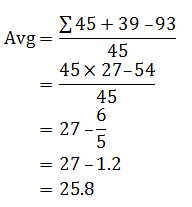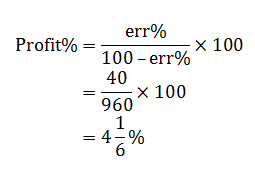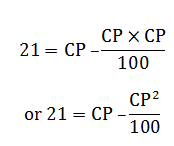or 2100 = 100 CP – CP²

or CP² – 100 CP + 2100 = 0

or (CP – 70) (CP – 30) = 0

or CP = 70,30

Percent increase in Area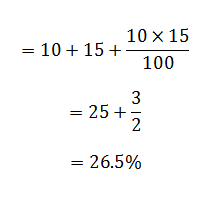% change in Area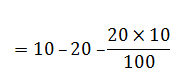= – 12%

∴ decreases in area by 12%

Let initial speed be x kmph.

So, a = x

d = 9 (common diff)

SU = 572 (sum)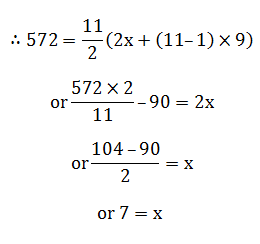Let the speed of boat = a km/hr

Let the speed of stream = x km/hr